# Ref vs Out in C#

## Ref vs Out Keywords in C# with Examples

In this article, I am going to discuss Ref vs Out Keywords in C# with Examples. Please read our previous article where we discussed the Volatile Keyword in C# with Examples. There are two keywords in C# i.e. Ref and Out and most of the developers are getting confused about these two keywords. So, at the end of this article, you will understand in what scenarios these keywords are useful and how to use them in C# with Examples.

##### Ref vs Out Keywords in C#:

The REF and OUT keywords in C# are used for passing the arguments to a method as a reference type. By default, arguments are passed to a method by value. By using these REF and OUT keywords in C#, we can pass arguments by reference. In this case, any changes made to these arguments in the method body will be reflected in those variable when the control returns to the calling method.

In order to understand the fundamental of both REF and OUT keywords in C#, please have a look at the following example. Here, you can see we have created one function called Math. This Math function takes two integer parameters and then this function adds these two numbers and returns the result. And from the Main method, we are invoking the Math function and then we are printing the result in the console that is returned by the Math function.

```using System;
namespace RefvsOutDemo
{
class Program
{
static void Main(string[] args)
{
int Result = Math(100, 200);
Console.WriteLine(\$"Result: {Result}");
}

public static int Math(int number1, int number2)
{
return number1 + number2;
}
}
}
```

Output: Result: 300

Now, my requirement is, when I call the Math function, I want to return the Addition, Multiplication, Subtraction, and Division of the two numbers passed to this function. But, if you know, it is only possible to return a single value from a function in C# at any given point in time i.e. only one output from a function.

If you look at the Math function, the return type is int which means it will only return a single value at any given point in time. Now, how we can return multiple values like the results of Addition, Multiplication, Subtraction, and Division of two numbers? So, in situations like this, we need to use out and ref parameters in C#.

##### Example using REF to Return Multiple Outputs From a Function in C#:

Now, let us first see how the ref keyword can help us to give multiple outputs from a function in C#. So, in order to return four values (Addition, Multiplication, Subtraction, and Division of the given two numbers) from the Math function, the Math function should accept four parameters apart from the two input parameters and the parameters should be declared with the ref keyword. And, then we need to set the values in these ref parameters as shown in the below code. Modify the Math function as follows. As we are returning the output using the ref parameter, so we changed the return type of this method to void.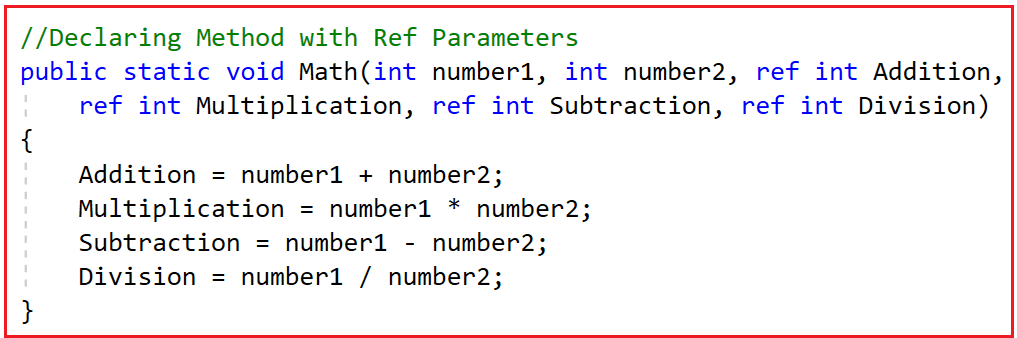Now, from the Main method, while calling the above Math function, apart from the two integer numbers, we also need to pass four integer ref arguments. To do so, first, we need to declare four integer variables. So here we declared four variables i.e. Addition, Multiplication, Subtraction, and Division. Then we need to pass these four variables to the Math function and the Math function will then give us the updated values for these variables. In order to get back the updated values into these variables, while passing these variables to the Math function, again, we need to use the ref keyword as shown in the below image.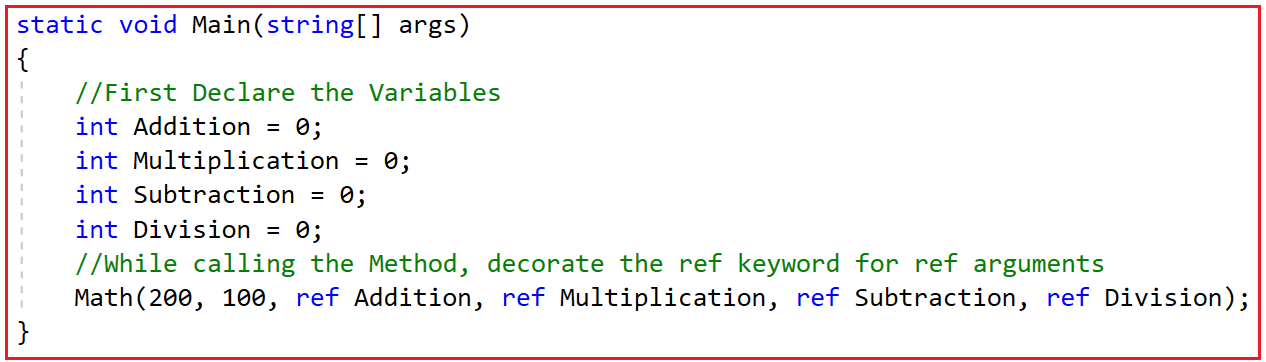Now, the Addition variable will hold the addition of the two numbers we passed to the Math function. Similarly, the Multiplication variable will give us the multiplication of the two numbers we passed to the Math function and the same for Division and Subtraction.

So, what actually happens is that when we update the ref variable inside the Math function, it will actually update the same inside the Main function. For example, if we update the Addition variable inside the Math function, it will actually update the Addition variable present inside the Main method. And the same for Multiplication, Subtraction, and Division. The complete example code is given below. The following example code is self-explained, so please go through the comment lines for a better understanding.

```using System;
namespace RefvsOutDemo
{
class Program
{
static void Main(string[] args)
{
//First Declare the Variables
int Multiplication = 0;
int Subtraction = 0;
int Division = 0;
//While calling the Method, decorate the ref keyword for ref arguments
//Addition, Multiplication, Subtraction, and Division variables values will be updated by Math Function
Math(200, 100, ref Addition, ref Multiplication, ref Subtraction, ref Division);

Console.WriteLine(\$"Multiplication: {Multiplication}");
Console.WriteLine(\$"Subtraction: {Subtraction}");
Console.WriteLine(\$"Division: {Division}");

}

//Declaring Method with Ref Parameters
public static void Math(int number1, int number2, ref int Addition,
ref int Multiplication, ref int Subtraction, ref int Division)
{
Addition = number1 + number2; //This will Update the Addition variable Declared in Main Method
Multiplication = number1 * number2; //This will Update the Multiplication variable Declared in Main Method
Subtraction = number1 - number2; //This will Update the Subtraction variable Declared in Main Method
Division = number1 / number2; //This will Update the Division variable Declared in Main Method
}
}
}
```
###### Output: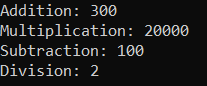So, you can observe here, by using the ref parameter how we are able to get multiple outputs from a single function in C#.

##### Important Notes:

Here, we are passing the parameter are value types. That means int, float, Boolean, etc. are used to create value-type variables. We already know the concept of Call by Value Mechanism in C#. In the case of value type, a different copy of the variables is passed to the calling method. If you do any changes in the Calling method, it will not affect the same original variables. But because we are using the ref keyword here before the argument name, it is actually passing a pointer here which will point to the original variables. So, changing the values using a pointer is actually changing the values of the original variables. This is nothing but Call By Reference Mechanism in C#.

##### Example using Out Parameter to Return Multiple Outputs from a Function in C#:

Let us first see the example first and then we will understand the concept of the OUT parameter in C#. Please have a look at the following example. This is the same example as the previous one, except instead of ref, we are using the OUT keyword here.

```using System;
namespace RefvsOutDemo
{
class Program
{
static void Main(string[] args)
{
//First Declare the Variables
int Multiplication = 0;
int Subtraction = 0;
int Division = 0;
//While calling the Method, decorate the out keyword for out arguments
//Addition, Multiplication, Subtraction, and Division variables values will be updated by Math Function
Math(200, 100, out Addition, out Multiplication, out Subtraction, out Division);

Console.WriteLine(\$"Multiplication: {Multiplication}");
Console.WriteLine(\$"Subtraction: {Subtraction}");
Console.WriteLine(\$"Division: {Division}");

}

//Declaring Method with out Parameters
public static void Math(int number1, int number2, out int Addition,
out int Multiplication, out int Subtraction, out int Division)
{
Addition = number1 + number2; //This will Update the Addition variable Declared in Main Method
Multiplication = number1 * number2; //This will Update the Multiplication variable Declared in Main Method
Subtraction = number1 - number2; //This will Update the Subtraction variable Declared in Main Method
Division = number1 / number2; //This will Update the Division variable Declared in Main Method
}
}
}
```
###### Output: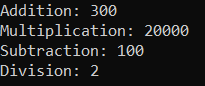Fine. We are getting the same result. That means using out, we are also getting the updated values from the Math function. So, it is working very similarly to the ref parameter. Now, the most frequently asked interview question is what are the differences between out and ref in C#?

##### What are the Differences Between OUT and REF Keyword in C#?

So, the first point that you need to remember is when you want multiple outputs from a function, then you need to use the ref and out parameters in C#. If you look out and ref, both are closely doing the same thing. Then what are the differences between them? Let us understand the differences with an example. Please have a look at the following example. The following is the code that we have already explained in our previous two examples.

```using System;
namespace RefvsOutDemo
{
class Program
{
static void Main(string[] args)
{
//Calling the Method with the REF arguments
int SubtractionRef = 0;
MathRef(200, 100, ref AdditionRef, ref SubtractionRef);
Console.WriteLine(\$"SubtractionRef: {SubtractionRef}");

//Call the Method with the OUT arguments
int SubtractionOut = 0;
MathOut(200, 100, out AdditionOut, out SubtractionOut);
Console.WriteLine(\$"SubtractionOut: {SubtractionOut}");

}

//Creating Method with Ref Parameters
public static void MathRef(int number1, int number2, ref int Addition, ref int Subtraction)
{
Addition = number1 + number2; //This will Update the Addition variable inside the Main method
Subtraction = number1 - number2; //This will Update the Subtraction variable inside the Main method
}

//Creating Method with out Parameters
public static void MathOut(int number1, int number2, out int Addition, out int Subtraction)
{
Addition = number1 + number2; //This will Update the Addition variable inside the Main method
Subtraction = number1 - number2; //This will Update the Subtraction variable inside the Main method
}
}
}
```
###### Output: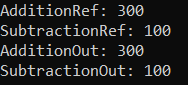Fine. Getting the output as expected.

##### Difference1: Updating the Ref and Out variables Inside the Method

When we call a method with the “out” variable, the method has to update the out variable inside the function and it is mandatory. But this is not mandatory if you are using the ref variable. For example, please have a look at the below code. Here, we are commenting on the second update statement inside both MathRef and MathOut functions. If you notice inside the MathRef function, we are not getting any compile time errors. But inside the MathOut method, we are getting one compile time error saying “The out parameter ‘Subtraction’ must be assigned to before control leaves the current method” as shown below.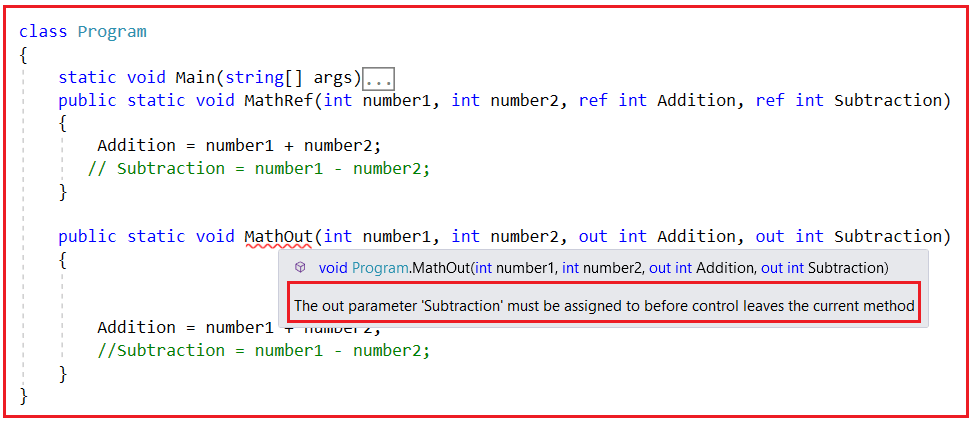So, the first point that you need to keep in mind is that, if you are declaring some out variables, then it is mandatory or compulsory to initialize or update the out variables inside the method body else we will get a compiler error. But with the ref, updating the ref variable inside a method is optional.

##### Difference2: Initializing the Ref and Out variables while passing to the Method

When we are passing the ref parameter as arguments, it is mandatory to initialize the ref parameter before passing it to the method else we will get compile time error. This is because with the ref parameter, updating the value inside the method is optional. So, before passing the ref parameter, it should be initialized. On the other hand, initializing an out parameter is optional. If you are not initializing the out parameter, no problem, because the out parameter is compulsorily initialized or updated inside the method. For a better understanding, please have a look at the below code. Here, we are not initializing the second parameter. For the SubtractionOut parameter, we are not getting any error, but for SubtractionRef, we are getting a compiler error saying Use of unassigned local variable ‘SubtractionRef’ as shown below.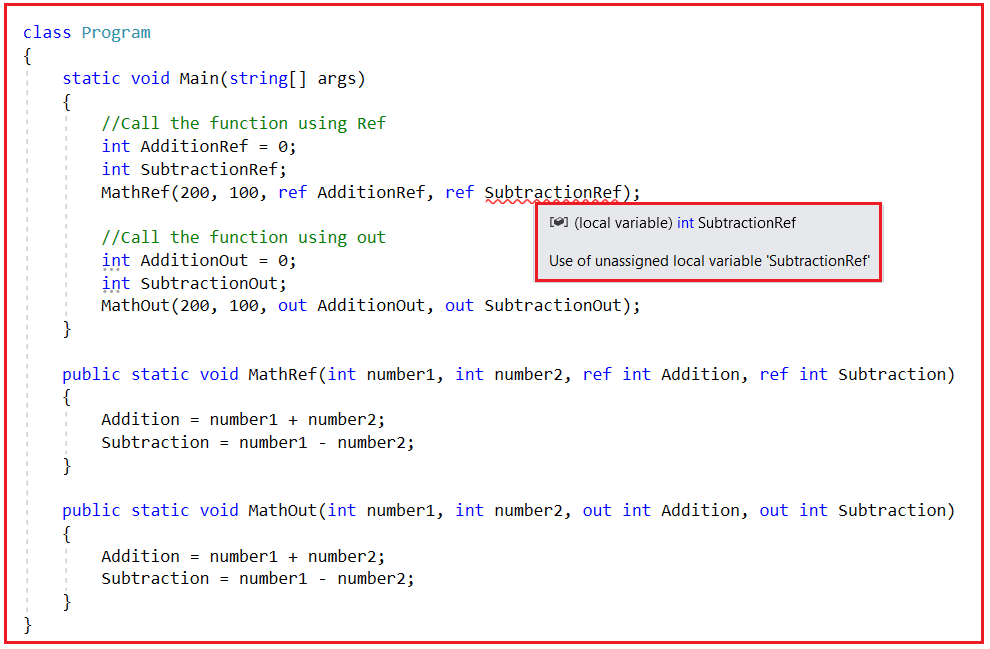So, the second important point that you need to keep in mind is that initializing the ref parameter is mandatory before passing such variables to the method while initializing the out-parameter variables is optional in C#.

Note: The point that you need to remember is that the ref keyword is used to pass data pass in bi-directional and the out keyword is used to pass the data only in unidirectional i.e. returning the data.

##### When to use REF Parameters in C#?

You need to use the ref parameters when you want to pass some values to the function and you expect the values to be modified or updated by the function and give you back. To understand this better, please have a look at the below example. Here, we have one function called AddTen. This function takes one integer parameter and increments its value by 10. So, in situations like this, you need to use the ref parameter. So, you are passing some value and you expect that value to be modified by the function.

```using System;
namespace RefvsOutDemo
{
class Program
{
static void Main(string[] args)
{
//Use of Ref in C#
int Number = 10;
Console.WriteLine(Number);
}

public static void AddTen(ref int Number)
{
Number = Number + 10;
}
}
}
```

In C#, you need to use ref when you have some values and you want those values to be modified by the calling function and give you back those modified values.

##### When to use the OUT Parameter in C#?

With the OUT parameter, we are only expecting the output from the method. We don’t want to give any input. So, we need to use the out parameter, when we don’t want to pass any value to the function and we expect the function should and must update the variable and return the value. For a better understanding, please have a look at the below example. Here, we are passing two integer numbers to the Add function and we expect the Add function to update the Result out parameter.

```using System;
namespace RefvsOutDemo
{
class Program
{
static void Main(string[] args)
{
//Use of out in C#
int Result;
Console.WriteLine(Result);
}

public static void Add(int num1, int num2, out int Result)
{
Result = num1 + num2;
}
}
}
```
##### Changes to OUT Parameter in C# 7:

The Out Parameter in C# never carries value into the method definition. So, it is not required to initialize the out parameter while declaring. So, here initializing the out parameter is useless. This is because the out parameter is going to be initialized by the method. Then you may have one question on your mind, if it is not required to initialize the out variables then why should we split their usage into two parts? First, declare the variable and then pass the variable to the function using the ref keyword.

With the introduction of C# 7, now it is possible to declare the out parameters directly within the method. So, the above program can be rewritten as shown below and also gives the same output. Here, you can see that we are directly declaring the variable at the time of the method call i.e. Add(10, 20, out int Number);. This will eliminate the need to split the usage of the C# out variable into two parts.

```using System;
namespace RefvsOutDemo
{
class Program
{
static void Main(string[] args)
{
//Use of out in C#
Console.WriteLine(Number);
}

public static void Add(int num1, int num2, out int Result)
{
Result = num1 + num2;
}
}
}
```

### 4 thoughts on “Ref vs Out in C#”

1.hello,

in the last two screenshots you have wrong comments though (says “Use of Ref in C#” but in those two examples you are using out keywords).

Ronald

1.Hi
Thanks for identifying the typo error. We have corrected the same.

2.thanks for sharing deep use of ref and out keywords.

3.hi, thanks for this very good tutorial, truly a step by step and clear run through, i went over a good few articles and left more puzzled after each one, until I read yours. Exactly how code should be explained.